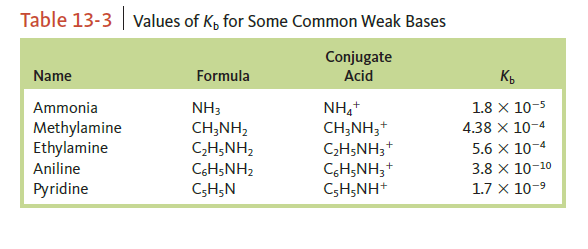# Problem: Calculate [OH-], [H+], and the pH of 0.40 M solution of the following amine (the K  b values are found in the following table).a. aniline

###### FREE Expert Solution

For this problem, we are being asked to calculate the pH, H+, and OH- concentration of 0.40 M Aniline

Establish the Kb expression of the dissolution of aniline and determine the pH using an ICE chart

We will solve this problem through these steps:

Step 1: Write an equation for the dissociation of aniline

Step 2: Write an expression for the Kb

Step 3: Set up an ICE table to calculate the OHion concentration.

Step 4: Calculate the pH of solution using OH- ion concentration.

Step 1: Dissociation of aniline in solution.

NaHCOO is a weak base and dissociates partially in aqueous solution.

C6H5NH2 (aq) + H2O(l) C6H5NH3+ (aq) + OH-(aq)                Kb = 3.8x10-10

Step 2: Kb expression for NaHCOO

The expression of Kb can be written like the equilibrium expression:

Solids and liquids are not included in equilibrium expressions.

99% (114 ratings)###### Problem Details

Calculate [OH-], [H+], and the pH of 0.40 M solution of the following amine (the K  b values are found in the following table).

a. anilineFrequently Asked Questions

What scientific concept do you need to know in order to solve this problem?

Our tutors have indicated that to solve this problem you will need to apply the Weak Bases concept. If you need more Weak Bases practice, you can also practice Weak Bases practice problems.

What textbook is this problem found in?

Our data indicates that this problem or a close variation was asked in Chemistry: An Atoms First Approach - Zumdahl Atoms 1st 2nd Edition. You can also practice Chemistry: An Atoms First Approach - Zumdahl Atoms 1st 2nd Edition practice problems.